# How to get Net Present Value Calculator in Microsoft Excel 2010

In this article, we will learn How to get a Net Present Value Calculator in Microsoft Excel 2010.

Net Present value

The Net Present Value (NPV) returns the net present value of an investment based on periodic, constant payments and a constant interest rate.

Net present value is calculated using a discount rate (which may represent an interest rate or the rate of inflation) and a series of future payments (negative values) and income (positive values).

NPV function Syntax :

 =NPV (rate, value1, value2, ….)

Arguments

Rate is the periodic discount rate over the length of the project

Value1, Value2, ……..Value n will be the value of the specific period on which calculation is based.

Value1 and Value2 are 1 to 29 arguments representing the payments and income. These value sets must be equally spaced in time and occur at the end of each period.

Let us understand more with an example:

Let’s use it in an example to understand it.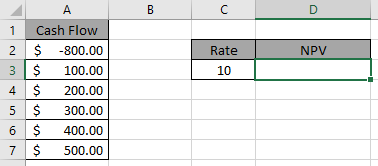Here one amount is debited and other amounts are credited at an interest of 10%.

Use the formula in D3 cell.

 =NPV(C3, A3:A7)+A2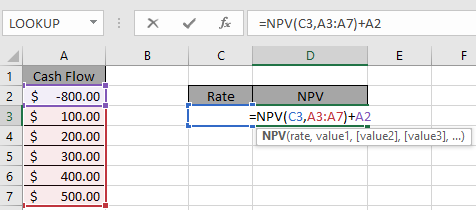Press Enter.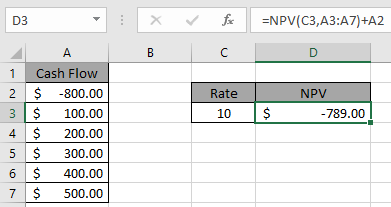It means the net present value of the whole expense is \$789.

This amount is to be debit.

Let us consider another example we have invested \$100,000 in a machine.The additional cash inflows (net income + depreciation) from the machine will be \$55,000, \$ 65,000, and \$80,000 over the next three years. Interest Rate is 6%. We want to calculate the Net Present Value (NPV)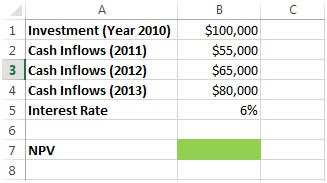• Formula we use in cell B7 =NPV(B5,B2,B3,B4)-100000
• We will get the below mentioned result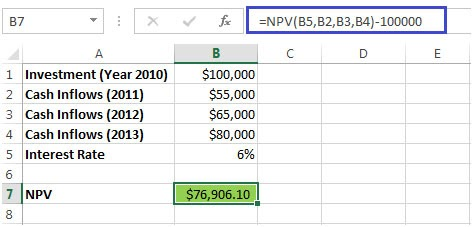• We have subtracted the Initial Investment to get the real NPV

Let us take another example

• Consider an investment of \$ 50,000
• 32,000, 35,000 & 28,000 are the figures that show the Income of the first three years respectively.
• On the basis of 10% Discount Rate, we want to find out the Net Present Value (NPV)
•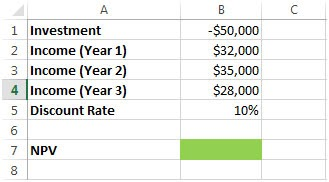• We will enter NPV formula in cell B7=NPV(B5,B1,B2,B3,B4)
• We will get the desired result
•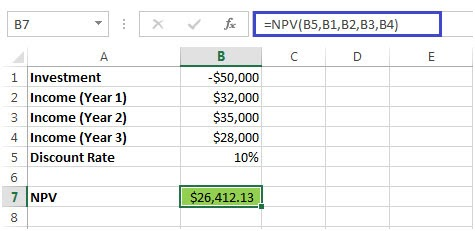Value in cell B5 is the Discount Rate
• Cell B1 is the Investment
• B2, B3 & B4 show the Income for every year.

Here are some observational notes shown below.

Notes:

1. Type argument is not default in this function, throws an error "you've entered too few arguments for this function", If argument is not used.
2. The formula only works with numbers only, returns #VALUE! Error if any argument is non-numeric.
3. Do not use negative numbers in the argument as the formula generate #NUM! Error.
4. If you make monthly payments on a four-year loan at an annual interest rate of 12 percent, use rate/12 for monthly rate. Use rate directly 12%, if calculating for annual payments
5. If the start period < 1, end period < 1 or Start period > end period, the formula returns #NUM! Error.
6. Type argument other than 0 or 1, the formula returns #NUM! error.

Hope you understood How to get Net Present Value Calculator in Microsoft Excel 2010. Explore more articles here on calculating financial values using functions here. If you liked our blogs, share it with your friends on Facebook. And also you can follow us on Twitter and Facebook. We would love to hear from you, do let us know how we can improve, complement or innovate our work and make it better for you. Write us at info@exceltip.com

Related Articles

Calculate the principal payment on cumulative loan using CUMPRINC function in Excel : cumulative principal paid based on Loan amount with constant interest rate given over a  period of time calculated using CUMPRINC function in Excel.

Simple interest formula in Excel : Calculate the simple interest amount given the present or principal amount, rate in annum & period in years.

How to calculate interest on a loan : monthly instalment on a loan amount using the PMT function having principal amount or loan amount, interest rate per month and the period of payment.

How to use the RATE Function in Excel : RATE function is used to find the Interest rate of the data set in Excel. It requires the following arguments to calculate the interest rate.

How to use the PV function in Excel : PV function returns the present value of the fixed amount paid over a period of time at a constant interest rate.

How to use the NPV function in Excel : NPV function is used to find the net present value of the data set in Excel.

How to use the FV function in Excel : FV function in Excel returns the future value of the present amount having interest rate over a period.

Popular Articles:

50 Excel Shortcut to Increase Your Productivity : Get faster at your task. These 50 shortcuts will make you work even faster on Excel.

How to use the COUNTIF function in Excel : Count values with conditions using this amazing function. You don't need to filter your data to count specific values. Countif function is essential to prepare your dashboard.

How to use the SUMIF Function in Excel : This is another dashboard essential function. This helps you sum up values on specific conditions.

How to use the VLOOKUP Function in Excel : This is one of the most used and popular functions of excel that is used to lookup value from different ranges and sheets.

Terms and Conditions of use

The applications/code on this site are distributed as is and without warranties or liability. In no event shall the owner of the copyrights, or the authors of the applications/code be liable for any loss of profit, any problems or any damage resulting from the use or evaluation of the applications/code.